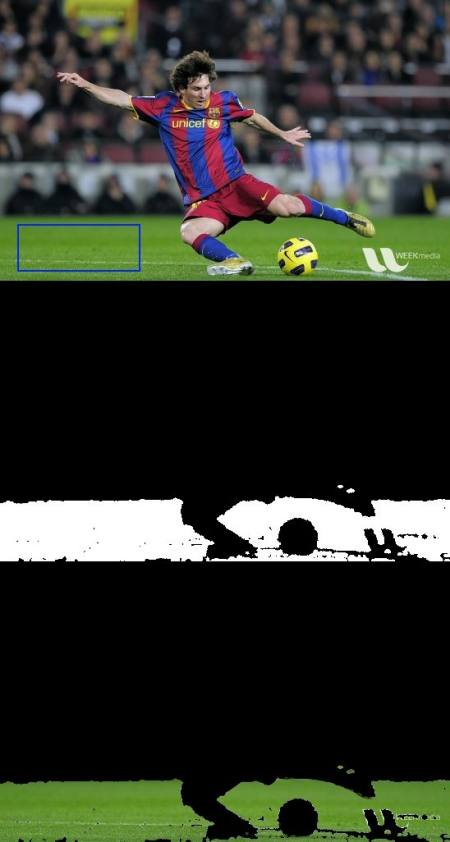# 直方图4：直方图反投影¶

### Numpy中的算法¶

1. 首先，我们需要计算我们要查找的对象（使其为“ M”）和要搜索的图像（使其为“ I”）的颜色直方图。
import numpy as np
import cv2 as cvfrom matplotlib import pyplot as plt
#roi是我们需要找到的对象或对象区域
hsv = cv.cvtColor(roi,cv.COLOR_BGR2HSV)
#目标是我们搜索的图像
hsvt = cv.cvtColor(target,cv.COLOR_BGR2HSV)
# 使用calcHist查找直方图。也可以使用np.histogram2d完成
M = cv.calcHist([hsv],[0, 1], None, [180, 256], [0, 180, 0, 256] )
I = cv.calcHist([hsvt],[0, 1], None, [180, 256], [0, 180, 0, 256] )

1. 求出比值$R = \frac{M}{I}$。然后反向投影R，即使用R作为调色板，并以每个像素作为其对应的目标概率创建一个新图像。即B(x,y) = R[h(x,y),s(x,y)] 其中h是色调，s是像素在(x，y)的饱和度。之后，应用条件$B(x,y) = min[B(x,y), 1]$
h,s,v = cv.split(hsvt)
B = R[h.ravel(),s.ravel()]
B = np.minimum(B,1)
B = B.reshape(hsvt.shape[:2])

1. 现在对圆盘应用卷积，$B = D \ast B$，其中D是圆盘内核。
disc = cv.getStructuringElement(cv.MORPH_ELLIPSE,(5,5))
cv.filter2D(B,-1,disc,B)
B = np.uint8(B)
cv.normalize(B,B,0,255,cv.NORM_MINMAX)

1. 现在最大强度的位置给了我们物体的位置。如果我们期望图像中有一个区域，则对合适的值进行阈值处理将获得不错的结果。
ret,thresh = cv.threshold(B,50,255,0)


### OpenCV的反投影¶

OpenCV提供了一个内建的函数**cv.calcBackProject**()。它的参数几乎与**cv.calchist**()函数相同。它的一个参数是直方图，也就是物体的直方图，我们必须找到它。另外，在传递给backproject函数之前，应该对对象直方图进行归一化。它返回概率图像。然后我们用圆盘内核对图像进行卷积并应用阈值。下面是我的代码和结果:

import numpy as np
import cv2 as cv
hsv = cv.cvtColor(roi,cv.COLOR_BGR2HSV)
hsvt = cv.cvtColor(target,cv.COLOR_BGR2HSV)
# 计算对象的直方图
roihist = cv.calcHist([hsv],[0, 1], None, [180, 256], [0, 180, 0, 256] )
# 直方图归一化并利用反传算法
cv.normalize(roihist,roihist,0,255,cv.NORM_MINMAX)
dst = cv.calcBackProject([hsvt],[0,1],roihist,[0,180,0,256],1)
# 用圆盘进行卷积
disc = cv.getStructuringElement(cv.MORPH_ELLIPSE,(5,5))
cv.filter2D(dst,-1,disc,dst)
# 应用阈值作与操作
ret,thresh = cv.threshold(dst,50,255,0)
thresh = cv.merge((thresh,thresh,thresh))
res = cv.bitwise_and(target,thresh)
res = np.vstack((target,thresh,res))
cv.imwrite('res.jpg',res)### 附加资源¶

1. “Indexing via color histograms”, Swain, Michael J. , Third international conference on computer vision,1990.